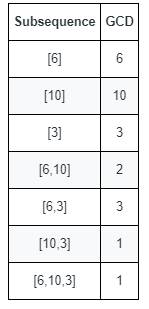# GeetCode Hub

You are given an array `nums` that consists of positive integers.

The GCD of a sequence of numbers is defined as the greatest integer that divides all the numbers in the sequence evenly.

• For example, the GCD of the sequence `[4,6,16]` is `2`.

A subsequence of an array is a sequence that can be formed by removing some elements (possibly none) of the array.

• For example, `[2,5,10]` is a subsequence of `[1,2,1,2,4,1,5,10]`.

Return the number of different GCDs among all non-empty subsequences of `nums`.

Example 1:```Input: nums = [6,10,3]
Output: 5
Explanation: The figure shows all the non-empty subsequences and their GCDs.
The different GCDs are 6, 10, 3, 2, and 1.
```

Example 2:

```Input: nums = [5,15,40,5,6]
Output: 7
```

Constraints:

• `1 <= nums.length <= 105`
• `1 <= nums[i] <= 2 * 105`

class Solution { public int countDifferentSubsequenceGCDs(int[] nums) { } }